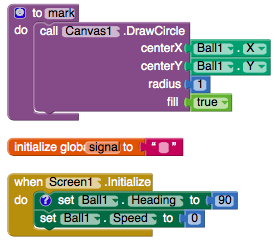# Rexplorer v2

Rexplorer v2 is a fully autonomous self-mapping robot to be used for indoor purposes.

My original intention was to develop a robot which could map an unknown area and relay the data to a monitoring system so as to provide real-time feedback about the layout of external environment.

I ended up making a robot that maps its own path, generally sticks to moving along the periphery of a room and sends a rough sketch of its traversed path to a smartphone via bluetooth.

This robot is part of the Rexplorer Project and is only an experimental prototype. There are certain conditions for sensing which have not yet been included, due to which undesirable movement may be caused. (Bug-fixes for the same will feature in the next version along with improved sensing algorithms and ultrasonic sensors.)

How it works:

There are 3 infrared proximity sensors at the front and sides of the robot which detect obstructions in its path. These digital signals are sent to an AVR micro-controller (ATMEGA 328 on Arduino UNO) which decides in which direction the robot should turn. The decision then activates the respective motors by sending a set of signals to the motor driver. Simultaneously, a second signal is sent to an Android application on a smartphone, via Bluetooth, containing information about the robot’s current movement which is in turn, used to draw a sketch of its path as it moves in real-time.

The Android application was made using MIT App Inventor 2 and the robot’s program was coded in the Arduino IDE (Integrated Development Environment), based on C++.

List of components: (all components are available at online stores such as robokits.co.in)

• Wheels – 4 nos.
• DC Geared motors – 4 nos.
• Arduino UNO – 1 no. (Any version of Arduino can be used with the given code, provided it has the same or greater number of I/O pins and relevant changes are made in the uploading procedure. Additionally, any development board can be used provided the code’s logic remains the same and sufficient I/O pins are available.)
• HC-05 bluetooth module – 1 no.
• Infrared proximity sensors – 3 nos.
• L298N motor driver – 1 no.
• Lithium-ion rechargeable battery – 1 no.
• Jumper wires – lots!

And finally, the codes! As always, please feel free to utilise, remix and improvise upon the codes. (And let me know how it went in the comments below!)

Smartphone application –Arduino code –

````int l1 = 6;` `int l2 = 5;` `int r1 = 11;` `int r2 = 9;` `int rs = 100;` `int ls = 100;` `int rts = 130;` `int lts = 130;` `//Coded by Raunak Hede-http://raunakhede.com` `//drive system` `#define f analogWrite(l1, ls); analogWrite(l2, 0); analogWrite(r1, rs); analogWrite(r2, 0);` `#define b analogWrite(l1, 0); analogWrite(l2, ls); analogWrite(r1, 0); analogWrite(r2, rs);` `#define l analogWrite(l1, 0); analogWrite(l2, lts); analogWrite(r1, rts); analogWrite(r2, 0);` `#define r analogWrite(l1, lts); analogWrite(l2, 0); analogWrite(r1, 0); analogWrite(r2, rts);` `#define s analogWrite(l1, 0); analogWrite(l2, 0); analogWrite(r1, 0); analogWrite(r2, 0);` `#define d delay(200);` `//individual hemisphere control` `#define leftfor analogWrite(l1, lts); analogWrite(l2, 0);` `#define rightfor analogWrite(r1, rts); analogWrite(r2, 0);` `#define leftstop analogWrite(l1, 0); analogWrite(l2, 0);` `#define rightstop analogWrite(r1, 0); analogWrite(r2, 0);` `#define leftback analogWrite(l1, 0); analogWrite(l2, lts);` `#define rightback analogWrite(r1, 0); analogWrite(r2, rts);` `//MAPPING system` `#define forward Serial.write("1");` `#define backward Serial.write("4");` `#define left Serial.write("3");` `#define right Serial.write("2");` `#define forright Serial.write("1");Serial.write("2");` `#define backright Serial.write("1");Serial.write("2");` `#define forleft Serial.write("4");Serial.write("3");` `#define backleft Serial.write("4");Serial.write("3");` `#define stp Serial.write("0");` `int state;` `int s1,s2,s3;` `void setup()` `{` `pinMode(l1, OUTPUT);` `pinMode(l2, OUTPUT);` `pinMode(r1, OUTPUT);` `pinMode(r2, OUTPUT);` `pinMode(A0, INPUT);` `pinMode(A2, INPUT);` `pinMode(A3, INPUT);` `Serial.begin(9600); //initialize serial communication at 9600 bits per second` `pinMode(13,OUTPUT);digitalWrite(13,LOW);` `}` `void loop()` `{` ` if(Serial.available()>0)` ` {` ` int fse=digitalRead(A0);//front` ` int lse=digitalRead(A2);//digital` ` int rse=digitalRead(A3);//digital` `//specify sensor limits` ` int fsl=0;` ` int lsl=0;` ` int rsl=0;` `//sets to 1 if closed and to 0 if open` ` if(lse==lsl){s1=1;}else{s1=0;}` ` if(fse==fsl){s2=1;}else{s2=0;}` ``` if(rse==rsl){s3=1;}else{s3=0;}
``` ` if(s1==0 && s2==0 && s3==0)` ` {//no blocks` ` forward;` ` f;delay(100);` ` }` ` else if(s1==1 && s2==0 && s3==1)` ` {//sides blocked` ` forward;` ` f;delay(100);` ` }` ` else if(s1==1 && s2==1 && s3==1)` ` {//all blocked- to rev- add about turn` ` stp;` ` s;` ` }` ` else if(s1==1 && s2==1 && s3==0)` ` {//turn 90 right` ` left;` ` r;delay(400);` ` }` ` else if(s1==0 && s2==1 && s3==1)` ` {//turn 90 left` ` left;` ` l;delay(400);` ` }` ` else if(s1==0 && s2==0 && s3==1)` ` {//wall on right` ` forward;` ` rightfor;leftstop;` ` }` ` else if(s1==1 && s2==0 && s3==0)` ` {//wall on left` ` forward;` ` leftfor;rightstop;` ` }` ` //T-junctions` ` else if(s1==0 && s2==1 && s3==0)` ` {// front block- turn 90 right` ` right;` ` r;delay(400);` ` }` ` }` ` else{s;}` `}````